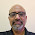Monday, March 12, 2018

#PythaShastri has Ramanujan type formula now

Remember, the formula for pi in Becoming Extroverted blog.
The formula was (2^(n(n+1)/2))/((n!)^(n-1)). I had given the explanations too.
It is tough to work like sun. So to say. Without emotion, without concern for others and doing your own thing. However, if you do it you get a function for pi. The above is the formula.
Now,
2^(n(n-1)/2) can be represented as C^n/(An+B). If I put this to the above equation, I will get an expression similar to pi of Ramanujan. I shall be working on this.
This was the last frontier.
So, Ramanujan famous pi formula can be compared.
Namaskarams, Ramanujan Sir in heaven. You did our country proud.

1.Final frontier should be group working (as I understand) pi.

2.I feel these guys/institutions are great, apart from Euler, Gauss and all.
1. Wolfram
2. eMathhelp.
3. Symbolab (I wish to make an Indian math widget for series)

3.Mathematician Abel was probably great. From his expressions.(Writing from memory).

4.From India, I liked Yashvant Kanetkar very much. He is a software guy though. I watched his video tutorials.

5.Euler was the greatest, however.